## Abstract

Measurements of Global Positioning Satellite System receivers are affected by systematic offsets related to group and phase delays of the signal generation and processing chain. One of the important factors affecting the ionosphere Total Electron Content estimation accuracy is the hardware differential code biases inherited in both Global Positioning System satellites and receivers. The resulting code and phase biases depend on the transmission frequency and the employed signal modulation. An efficient algorithm using the geometry conditions between satellite and tracking receivers is proposed to determine the receiver differential code biases using Egyptian permanent reference stations. This method does not require a traditional single-layer ionosphere model and can be used for estimating differential code biases of receivers in a regional network.

This paper estimates receiver differential code biases for nine receivers located within Egyptian network. The results showed that the estimated mean value of the receiver differential code biases varied from −28 ns (nanosecond) to 39 ns. It is clear from the results that differential code biases values for Egyptian sites do not vary much with latitude and longitude, except at Aswan and Abu Simpel. Differential code biases values increase gradually with increasing height.

## Keywords

Global Positioning System; Differential code bias; Total Electron Content; Ionospheric delay

## 1. Introduction

Today Global Positioning System (GPS) is widely used for navigation and positioning in either static or kinematic mode in a number of applications. Ionospheric influence can reduce the accuracy of positioning by tens of meters. The ionospheric influence might reach more than a hundred meters during a violent ionospheric storm. This ionospheric effect has apparently become the largest error source in GNSS navigation and positioning after Selective Availability (SA) is turned-off for single-frequency users . Therefore, the ionospheric effects must be considered for high accuracy positioning.

The Total Electron Content (TEC) in the ionosphere can be easily estimated from the combination of the Global Positioning System (GPS) data. The derived TEC data by GPS measurements have an uncertainty because each GPS satellite transmitter and receiver hardware have associated biases that seriously affect the accuracy of the ionospheric TEC estimates  and .

In general, GPS TEC is calculated with the so-called geometry-free linear combination of two frequencies (L1–L2). Hardware biases usually remain in the ionosphere TEC after subtracting measurements at different frequencies. These differences in the hardware biases of GPS code measurements are called differential code biases (DCBs)  and . In other words, the differential code bias (DCB) is the differential hardware (e.g., the satellite or receiver) delay that occurs between two different observations obtained at the same or two different frequencies. The characteristics of the hardware delay are mainly related to the performances of corresponding instruments, and their values are different for each observation and each frequency. The DCB is actually treated in a relative sense, where the hardware delay of a given observation is used as the standard. DCB can be classified into two categories: the inter-frequency bias, which is the bias between observations at two different frequencies; and the intra-frequency bias, which is the bias between two observations at the same frequency .

The magnitude of the combined satellite and receiver DCBs can reach up to several nanoseconds (ns), one ns corresponds to approximately 30 cm in range units. To improve the accuracy of TEC estimates, it is necessary to precisely estimate GPS satellite and receiver DCBs. Usually, DCBs are estimated together with the ionosphere model; thus, there exists a high correlation between the estimated DCBs and the selected ionosphere model. According to Wilson and Mannucci , the TEC, when estimated from GPS measurements, may result in errors from ±3 ns to ±10 ns. One approach for receiver DCB estimation is suggested by determination of the receiver DCBs using a regional GPS network. However, one of the receiver DCBs needs to be set to an arbitrary reference value in order to avoid singularities in the parameter estimation process. Another approach is to estimate DCB for a single receiver at the zero difference level.

In this study, receiver DCB of an Egyptian Permanent GNSS Network (EPGN) is estimated using the single receiver approach. Data of about 36 days spanning a year are used. The variation of DCB with site and time is estimated to check the temporal and spatial DCB variation. The results indicate that DCB changes with time and site. The range of change is about −11 to −22 ns in the south part of Egypt, while in the north DCB values are about −9 to −16 ns.

## 2. Data

The used data were collected from a chosen part of EPGN. This part of EPGN is composed of nine dual frequency GPS receivers. The locations of these receivers are shown in Fig. 1. Table 1 shows the Cartesian coordinates of the nine stations. The network covers an area of about 947 km by 484 km in latitude and longitude approximately. Data are collected using two different Trimble model receivers, namely Trimble 5700 and Trimble NETR5. Both receivers provide non-correlated C1 and P2 observation data types. The data used were collected over a whole year, where every month was represented by three days. This makes thirty-six (36) days of data for the whole year. The original data were in Receiver Independent Exchange format (RINEX) format with one second sampling rate. These data were decimated to 30 s sampling rate before processing to reduce the number of equations. The elevation cut-off angle of 10° was used for the collected data. The precise ephemeris (SP3) and ionospheric models are taken from IGS.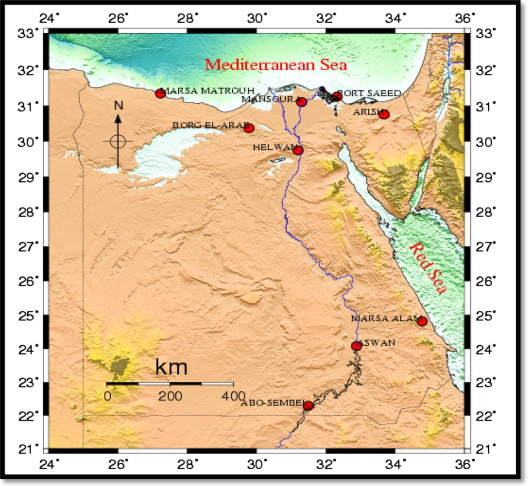Figure 1. Location of the used GPS station.

Table 1. Cartesian coordinates of the GPS sites.
Station Id. X (m) Y (m) Z (m)
PHLW 4728141.2348 2879662.6041 3157147.1275
MNSR 4671006.2282 2845893.5711 0269812.0787
SAID 4612664.2381 2917621.3718 3289234.9243
BORG 4765954.3185 2704546.1674 3252949.1622
ARSH 4551743.5738 3026108.3528 3276117.4525
MTRH 4847946.8930 2494773.3017 298721.2242
ASWN 4899061.5611 3163086.8817 2575414.1543
ABSM 5024945.1535 3084578.9769 2424694.7180
ALAM 4742516.3847 3305688.9798 2685814.2467

## 3. Data analysis

The DCB of a GPS receiver varies depending on the properties of the receiver and the observation type. Thus, for precise applications in geodesy and surveying, distinct DCBs for the various C1/P2 receivers should be derived from actual observations. As it is shown in Fig. 1, the data from the local nine GPS reference stations of EPGN were used for the calculation and analysis of the DCB over Egypt.

DCB is generally estimated once a day because space weather can affect DCB results and the daily variation of the receiver DCB is relatively stable. In this study, the receiver DCB values are estimated using the weighted Least Square Adjustment (LSA) and determined as daily value. The daily averages are obtained by taking the overall mean of the daily receiver DCBs over one day. The processing main steps are summarized as a block diagram in Fig 2. Starting with the Rinex files, the algorithm calculates the L4 and P4 linear combination. Also, receiver position is calculated. The P4 is then smoothed before LSA. Sp3 ephemeris is used to estimate the satellite positions. Receiver and satellite positions are then used to calculate the ionosphere pierce point (IPP). IPP and smoothed P4 are used in the LSA to estimate the DCBs.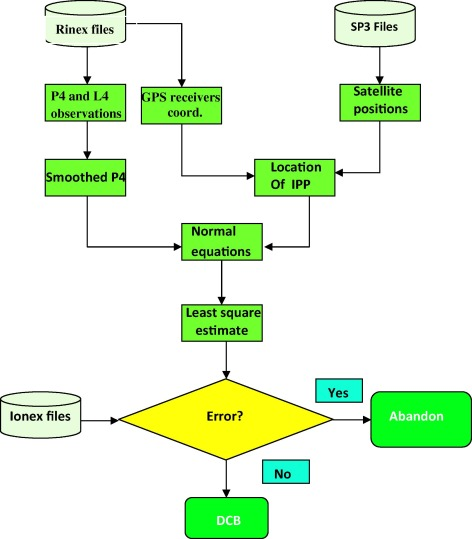Figure 2. Block diagram of the algorithm.

### 3.1. DCB algorithm description

The software was developed in MATLAB by Jin et al.  and revised and updated by Sedeek et al. . GPS RINEX observation files and precise ephemeris are the input data. Rinex files used here have C1 and P2 data. The outputs are the DCB estimates of the satellites and receivers. The revised software package can estimate the DCB for a single station or for multiple stations. For a single station, usually not all the GPS satellites are available in one GPS receiver view. It is not convenient to use the constraint condition for such a case. IONEX files are used to confirm our estimate using the same constraint conditions. The DCBs of satellites without observations are set as known parameters.

### 3.2. GNSS observations and pre-processing

GNSS observations include carrier phase and pseudorange observations stored in RINEX format. The GPS observation equations for pseudorange and carrier phase observables can be stated as follows (e.g. ):

 $P_{k{\mbox{,}}j}^{i}={\rho }_{j}^{i}+d_{ion{\mbox{,}}k{\mbox{,}}j}^{i}+$$d_{trop{\mbox{,}}j}^{i}+c({\tau }^{i}-{\tau }_{j})+d_{k}^{i}+d_{k{\mbox{,}}j}+$${\epsilon }_{p{\mbox{,}}k{\mbox{,}}j}^{i}$
(1)
 $L_{k{\mbox{,}}j}^{i}={\rho }_{j}^{i}-d_{ion{\mbox{,}}k{\mbox{,}}j}^{i}+$$d_{trop{\mbox{,}}j}^{i}+c({\tau }^{i}-{\tau }_{j})-\lambda (b_{k{\mbox{,}}j}^{i}+$$N_{k{\mbox{,}}j}^{i})+{\epsilon }_{L{\mbox{,}}k{\mbox{,}}j}^{i}$
(2)

where P is the GPS pseudorange measurement, L is the GPS carrier phase measurement, ρ is true distance between the GPS receiver (j) and satellite (i), dion is ionosphere delay, dtrop is troposphere delay, c is speed of light in a vacuum, τi is the satellite clock error, τj is the receiver clock error, the remaining d are the code delays for the satellite and receiver instrument biases, b is the phase advance of the satellite and receiver instrument biases, N   is the ambiguity of the carrier phase, and ${\textstyle \epsilon }$ are the residuals in the GPS measurements. Here, the subscript k (= 1, 2) stands for the frequency, the superscript i stands for the PRN of the GPS satellite, and the subscript j stands for the GPS receiver.

Using dual-frequency (fL1 = 1575.42 MH, fL2 = 1227.60 MHz) observations, the ionospheric delays can be estimated as follows:

 $P_{4}=P_{1{\mbox{,}}j}^{i}-P_{2{\mbox{,}}j}^{i}=(d_{ion{\mbox{,}}1{\mbox{,}}j}^{i}-$$d_{ion{\mbox{,}}2{\mbox{,}}j}^{i})+{DCB}^{i}+{DCB}_{j}$
(3)
 $L_{4}=L_{1{\mbox{,}}j}^{i}-L_{2{\mbox{,}}j}^{i}=-(d_{ion{\mbox{,}}1{\mbox{,}}j}^{i}-$$d_{ion{\mbox{,}}2{\mbox{,}}j}^{i})-\lambda (b_{1{\mbox{,}}j}^{i}-$$b_{2{\mbox{,}}j}^{i})-\lambda (N_{1{\mbox{,}}j}^{i}-N_{2{\mbox{,}}j}^{i})$
(4)

where DCBi = di1di2, and DCBj = d1,jd2,j stand for differential code biases of the satellites and differential code biases of the receivers, respectively. Since the pseudorange observations P4 have larger noise, the carrier phases are used to smooth the pseudorange. Then smoothed P4,sm observations is calculated using the following equations:

 $P_{4{\mbox{,}}sm}={\omega }_{t}P_{4}(t)+(1-{\omega }_{t})P_{4{\mbox{,}}prd}(t)\quad (t>1)$
(5)

where t stands for the epoch number, ωt is the weight factor related with epoch t and

 $P_{4{\mbox{,}}prd}(t)=P_{4{\mbox{,}}sm}(t-1)+[L_{4}(t)-L_{4}(t-$$1)]\quad (t>1)$
(6)

When t equal to 1 means the first epoch of one observation arc, P4,sm is equal to P4 after smoothing. Before using the carrier phase observations to smooth the pseudorange, Cycle slips and gross errors in the carrier phase observations should be detected and removed observations. To detect cycle slips and gross errors, both Melbourne–Wubeena combination and ionospheric residual observations are used. Due to the small effect of the higher ionospheric orders, only the first order of ionospheric refraction is considered while estimating the ionosphere delay in GPS processing. The ionosphere delay can be expressed as follows:

 $d_{ion}={\frac {40.3}{f^{2}}}STEC$
(7)

where f stands for the frequency of the carrier, and STEC stands for the Slant Total Electron Content along the path of the signal. Substituting (7) into (3) and using smoothed P4 (P4,sm) we get the following:

 $P_{4{\mbox{,}}sm}=40.3\left({\frac {1}{f_{1}^{2}}}-{\frac {1}{f_{2}^{2}}}\right)STEC+$${DCB}^{i}+{DCB}_{j}$
(8)

After smoothing, more reliable DCB estimate values can be extracted from GPS data as described in the next section.

### 3.3. DCB determination

Using Eq. (8), one can easily extract Slant Total Electron Content (STEC) from GNSS dual-frequency observations as follows:

 $STEC=-{\frac {f_{1}^{2}f_{2}^{2}}{40.3(f_{1}^{2}-f_{2}^{2})}}(P_{4{\mbox{,}}sm}-$${cDCB}_{j}-{cDCB}^{i})$
(9)

where DCB’s unit is the time. The earth’s ionosphere effective ranges in altitude are from 60 to 1000 km. All electrons in the ionosphere are assumed to be concentrated in a thin shell at altitude H, and hence the Vertical Total Electron Content (VTEC) can be calculated from STEC using the Mapping Function MF of the Modified Single-Layer Model , namely:

 $VTEC=MF(Z)STEC$

 $MF=cos\left(arcsin\left({\frac {R}{R+H}}sin(\alpha z)\right)\right)$
(10)

where ${\textstyle z}$ is the satellite elevation angle, R is the earth’s radius, and H is the altitude of the ionosphere thin shell (normally the approximate peak height of the F2 layer). R is set to 6371 km. H and α can be set by users. Here, they are defaulted as H = 506.7 km and α = 0.9782, which are consistent with the values used by the CODE group. The VTEC can be expressed using Taylor series expansions as given in Schaer :

 $E(\beta {\mbox{,}}s)=\sum _{n=0}^{n_{max}}\sum _{m=0}^{n}{\tilde {p}}_{nm}(sin\beta )(a_{nm}cosms+$$b_{nm}sinms)$
(11)

where E(βS) is the series expansion of VTEC, β is the geocentric latitude of the ionosphere pierce point (IPP), s = λλ0 is the sun-fixed longitude of the IPP, λ, λ0 are the longitude of the IPP and the apparent solar time, respectively, anm, bnm   are the global or regional ionosphere model coefficients, and ${\textstyle {\tilde {p}}_{nm}}$ = Λ(nm)Pnm are normalized Legendre polynomials. The Λ represent the normalization function, and Pnm are un-normalized Legendre polynomials, with the following:

 $\Lambda ={\sqrt {2{\frac {2n+1(n-m)!}{1+{\delta }_{0m}(n+m)!}}}}$
(12)

 $\sum _{n=0}^{n_{max}}\quad \sum _{m=0}^{n}{\tilde {p}}_{nm}(sin\beta )(a_{nm}cosms+$$b_{nm}sinms)=cos\left(arcsin\left({\frac {R}{R+H}}sin(\alpha z)\right)\right)-$${\frac {f_{1}^{2}f_{2}^{2}}{40.3(f_{1}^{2}-f_{2}^{2})}}(P_{4{\mbox{,}}sm}-$${cDCB}_{j}-{cDCB}^{i})$
(13)

where δ being the Kronecker Delta and P4,sm is the smoothed observations. The unknown parameters can be estimated: anm, bnm, DCBj and DCBi, by Substituting (9) and (10) into (11). The order of spherical harmonics expansion depends on the areas. The appropriate order in the main program(s) can be set by the user. From experience, for the regional, continental, and global network, 4th, 8th, and 15th order can be used, respectively. Here, for our EPGN case, the spherical harmonics expansion is defaulted as tenth order. A set of ionosphere coefficients is assumed every 2 h, but of course it can be changed. For one GPS station, there are about 20,000 measurements every day.

Based on the above discussion, DCB and ionosphere coefficients can be estimated from GPS dual-frequency observations by the least squares (LS) method. To overcome Eq. (13) singularity, one exterior constraint condition can be added in order to separate the DCBs of satellites and receivers. The sum of all GPS satellite DCB values is set to zero for this purpose. Under this constraint condition, the DCBs of the satellites and receivers can be separated.

## 4. Results and discussion

In order to test and validate the algorithm, Sedeek et al.  used data from IGS stations. Their results indicate a very good agreement between DCBs estimated by the algorithm used here and IGS estimated values available from the Internet. The results showed that the two algorithm differences are at the level of 5%.

### 4.1. The effect of station separation on computed DCB values

The DCB daily variations depend on how well the model is fitted with the VTEC spatial distributions. Therefore, if two stations are close to each other, the DCB variations should be highly correlated and the correlation will be reduced if the distance between two stations increases . Here, we selected a number of stations in Egypt, with distances from 94 (MNSR–SAID) to 1069 (MTRH–ABSM) km. A daily receiver DCB values as well as their mean for three days in each month during one year were computed. Additionally, for each receiver, the maximum DCB variations (maximum value–minimum value) and Standard Deviation (SD) value for the three-day period were calculated.

The results are shown in Table 2 which gives the daily variation range and SD of receiver DCB for all stations used here. When two stations are very close (94 km), the correlation is very high. This means that the DCB variation trends of these two stations are almost the same. On the other hand, with the increase in distance, the correlations decrease with distance. This test, from another point of view, demonstrates that estimated DCB variations are closely linked to the fitness of the ionospheric model to the real VTEC spatial distributions.

Table 2. The daily variation range and SD of receiver DCB of Egypt area.
Station name Lat. Long. DCB max. DCB min. Max. var. monthly SD Height (m)
PHLW 29.86 31.34 6.62 3.75 2.57 0.63 148.75
MNSR 31.04 31.35 −11.21 −12.17 −0.96 0.53 39.58
SAID 31.24 32.31 −10.95 −11.52 80.57 0.40 41.96
BORG 30.86 29.57 −9.06 −9.62 −0.56 0.36 098.09
ARSH 31.11 33.62 −25.15 −25.83 −0.68 0.37 027.41
MTRH 31.34 27.23 −8.76 −9.76 −1.00 0.50 58.68
ASWN 23.97 32.85 −19.44 −26.74 −7.30 3.93 215.51
ABSM 22.49 31.54 38.96 35.53 3.43 1.79 222.28
ALAM 25.07 34.88 −12.97 −12.10 0.87 0.43 48.88

The DCBs of the nine local permanent station receivers are shown in Fig. 3. Also indicated in the figure are the means of the nine receiver biases of different days. It should be pointed out that for the same season day to day variation is small. The DCB estimated in this region for the nine station can be categorized into four classes; the first one contains ASWN and ARSH, and the second contains SAID, MTRH, MNSR, BORG and ALAM stations, the third is PHLW station only and the forth is ABSM station. In the first class, namely ASWN and ARSH, ARSH DCB values vary between −25.41 and −32.61 ns and for Aswan the DCB values vary between −22.24 and −13.37 ns. As it is demonstrated in Fig. 4, the two stations are located in the opposite side of the study area: ASWN is in the south and ARSH in the north part of Egypt. The same receivers are used at ASWN, and ARSH station (Trimble NETR5). As it is depicted in the two columns of ASWN and ARSH in Table 3, one can easily see obvious transitions between different seasons for both receivers where the maximum differences between both seasons DCB values of both stations are occurred in the summer and winter. This is taken place because the maximum differences in weather temperature are occurred in both seasons.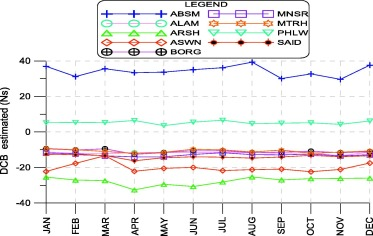Figure 3. C1–P2 differential code bias estimates for the GPS receivers.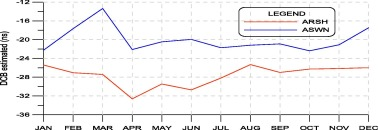Figure 4. C1–P2 differential code bias estimates for the GPS receivers (1st GROUP).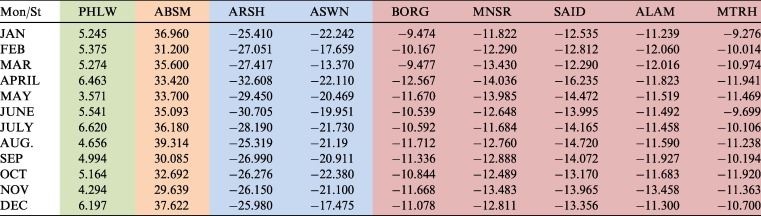The second group is BORG, MNSR, MTRH, SAID, and ALAM. The DCB values are about −11.24 ns to −13.46 at ALAM, SAID from −16.24 to −12.81 ns, MTRH range from −9.7 to −11.94 ns, MNSR from −11.82 to −14.04 ns, and BORG range from −9.48 to −12.57 ns as shown in Fig. 5. The mean standard deviation is 0.72 ns showing that receiver biases are more stable through this limit for the GPS satellites.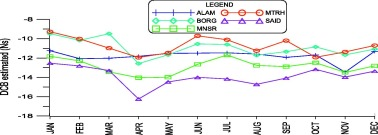Figure 5. C1–P2 differential code bias estimates for five local stations (2nd GROUP).

The third is PHLW station which is different from the other stations. Its DCB ranges from 3.57 to 6.62 ns as depicted in Fig. 6, so the DCB is positive and more stable than the other stations. The receiver used is Trimble5700 and this is the only station with this receiver type, while the other station used Trimble NETR5.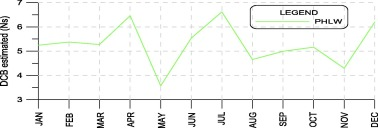Figure 6. C1–P2 differential code bias estimates for PHLW station (3rd GROUP).

On the other hand, for the fourth group ABSM, the DCB values are in the range of 39.31–29.64 ns. ABSM DCBs have bigger variations compared to the other stations’ DCB values. The reason of ABSM DCB high values and variations may be that its number of observation is much less than other stations. Also the magnitude of ABSM DCBs is positive see Fig. 7. From this result we can see that it is not clear why the bias of the receiver at ABSM is positive. The standard deviation in receiver bias for ABSM is 0.24–6.7 ns for the 36 days during 2014.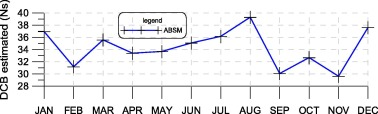Figure 7. C1–P2 differential code bias estimates for ABSM station (4th GROUP).

The above description on the results of the instrumental biases (DCB) shows that the algorithm is efficient and reliable for the quiet ionospheric conditions over northern Egypt in 2014 except ABSM station. Note that year 2014 is in the period of solar minimum when the ionospheric variability would be suppressed.

SD error values for each receiver DCB were computed. The corresponding SD values of DCBs range between 0.04 ns and 6.7 ns. In general, most of receivers of local reference stations in Egypt can be classified into two Trimble models (NETR5, Trimble 5700). The mean standard deviation (σ) of instrumental biases for receiver is presented in Table 4. The σ values indicate the monthly variability of the receiver differential delay estimates as in Fig. 8.

Table 4. The mean standard deviation (σ).
MON ABSM ALAM ARSH ASWN BORG MNSR MTRH PHLW SAID
JAN 1.786 0.784 0.404 0.371 3.935 0.362 0.66 0.633 0.527
FEB 6.697 1.401 0.224 0.188 0.164 0.226 1.164 0.091 0.64
MAR 1.77 0.042 0.04 0.736 0.826 0.83 0.489 0.522 0.17
APR 1.44 1.59 2.588 4.05 0.925 0.926 0.07 1.976 0.249
MAY 3.437 1.039 1.329 2.49 0.566 0.566 0.699 0.147 0.7
JUN 0.237 0.597 3.446 0.367 0.931 1.347 0.527 0.392 0.265
JUL 3.017 1.761 3.02 2.54 0.47 0.872 1.105 0.793 0.35
AUG 1.443 0.953 0.51 0.184 0.18 0.141 1.358 0.733 0.4
SEP 3.591 2.392 0.45 2.013 1.33 1.33 1.365 0.92 0.71
OCT 7.06 3.201 1.373 2.91 2.91 0.518 1.05 1.463 1.171
NOV 2.933 0.663 2.112 2.94 0.191 0.191 0.302 3.26 0.113
DEC 2.211 1.004 0.708 2.94 0.402 0.491 0.283 3.26 0.364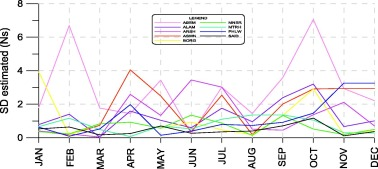Figure 8. Monthly SD error for estimated DCB.

As it is depicted in Fig. 9, the DCB for the local station, except ABSM at 31.5 Longitude, does not vary much with the longitude. Fig. 10 indicates that the DCB for the local station, except ABSM at 22.5 Latitude, is not changed much with the latitude. As it is depicted in Fig. 11, one can easily see that the height of the station affects the DCB values. The DCB gets higher gradually with increasing the height, except for ASWN at 220 m height.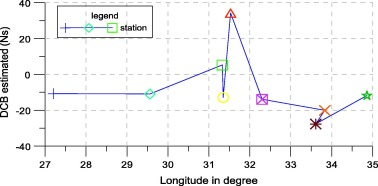Figure 9. DCB estimated with longitude.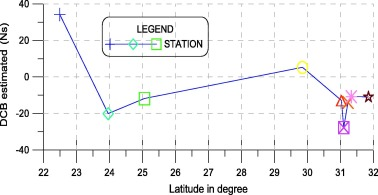Figure 10. DCB estimated with latitude.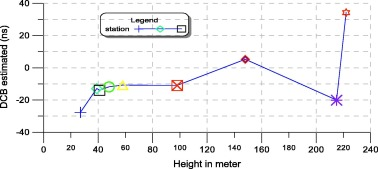Figure 11. DCB estimated with height.

## 5. Conclusions

With a chosen part of EPGN consists of nine GPS receivers located in Egypt, the current paper uses a DCB algorithm to determine the GPS receivers’ DCB biases. The method is applied to the GPS chain observations in four seasons of 2014. The results show clear diurnal day-to-day and seasonal variations, although similar to one another at different stations. The DCB and its temporal variations change with latitude and height.

The range of temporal change is from 39.31 to −22.38. The DCB changes with latitude but does not show a unique trend. DCBs increase gradually from −27.6 to 34.3 with height. Almost DCB does not show a clear trend of change with longitude. It is important to note that ABSM station DCB values are in the range of 29.6–39.3 ABSM DCB does not follow the latitude change model like the rest of the stations. ASWN Station has exceptional value which means that it does not obey the same height gradual increase like the remaining stations. The GPS receivers’ DCB biases are rather stable. The standard deviation is from 0.04 ns to 6.69 ns for the 36 days covering twelve-month time span. Standard deviation mean is 3.36 ns.

## Acknowledgments

We would like to express our sincere thanks to the staff of crustal movement Lab., Geodynamic department of NRIAG for providing GPS data used in the current research. Also, we would like to express our gratitude to the staff of public work department, civil Engineering, Mansoura University, for the good research environment. This research is supported by Tikrit University, the Iraqi Ministry of Higher Education, Baghdad, Republic of Iraq.

### Document informationPublished on 12/04/17

Licence: Other

### Document Score0

Views 28
Recommendations 0